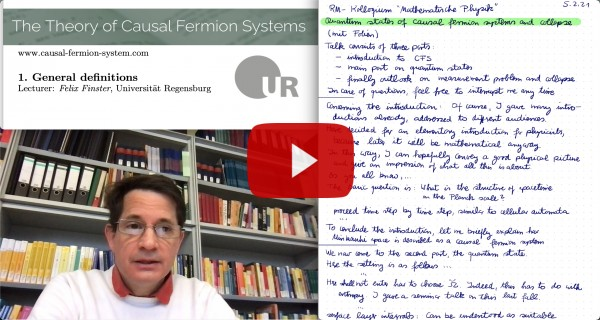# The Theory of Causal Fermion Systems

## Spin Spaces and Physical Wave Functions## The Spin Spaces

The causal action principle depends crucially on the eigenvalues of the operator product $xy$ with $x,y \in \F$. For computing these eigenvalues, it is convenient not to consider this operator product on the (possibly infinite-dimensional) Hilbert space $\H$, but instead to restrict attention to a finite-dimensional subspace of $\H$, chosen such that the operator product vanishes on the orthogonal complement of this subspace. This construction leads us to the spin spaces, which we now introduce. For every $x \in \F$ we define the spin space $S_x$ by $S_x = x(\H)$; it is a subspace of $\H$ of dimension at most $2n$ (for clarity, the spin space is often also denoted by $S_xM$). On the spin space one introduces the spin inner product $\Sl\! .|. \Sr_x$ by

$\Sl u |\; v \Sr_x : S_x \times S_x \longrightarrow \C\:,\qquad \Sl u |\: v \Sr_x = -\la u |\: x v \ra_\H \:.$

The spin space $(S_x, \Sl .|. \Sr_x)$ is an indefinite inner product space of signature $(p,q)$ with $p,q \leq n$.

In this way, to every spacetime point $x \in M$ we associate a complex vector space. This structure resembles a vector bundle. However, as a major difference to a bundle structure, here all the spin spaces are subspaces of one larger vector space, the Hilbert space $\H$. This is illustrated in the following figure:

## The Physical Wave Functions

A wave function $\psi$ is a mapping which to every spacetime point $x \in M$ associates a vector $\psi(x) \in S_x$ of the corresponding spin space. The vectors in the Hilbert space can be represented by specific wave functions, referred to as the physical wave functions: Given $u \in \H$, the corresponding physical wave function $\psi^u(x)$ evaluated at $x \in M$ is obtained by taking the orthogonal projecton on the spin space $S_x$,

$\psi^u \::\: M \longrightarrow \H \:,\qquad \psi^u(x) = \pi_x u \in S_x$

(where $\pi_x : \H \rightarrow S_x \subset \H$ is the orthogonal projection). This construction is illustrated in the following figure:

$SM := \bigcup_{x \in M} S_x \:,$
gives a structure similar to a vector bundle. The only difference is that the dimension of the fibres may depend on $x$. The physical wave functions give distinguished sections of this bundle. No smoothness assumptions are required. The topology of this so-called topological spinor bundles and the connection to the topological spin assumption of spin manifolds are worked out in [topology14].